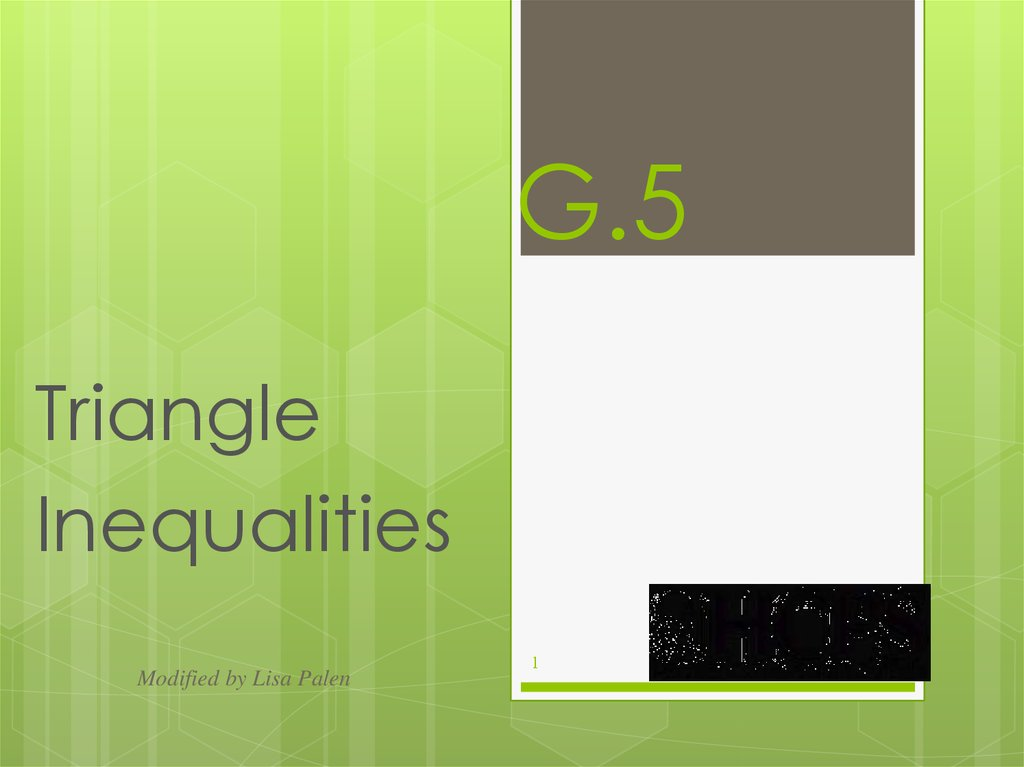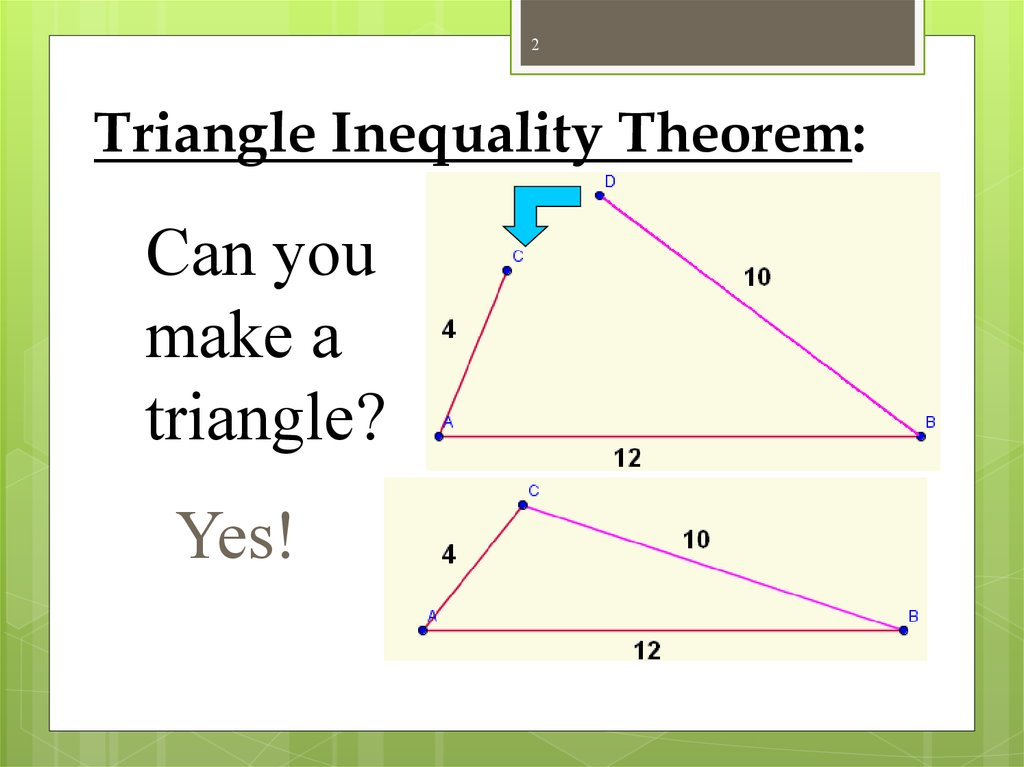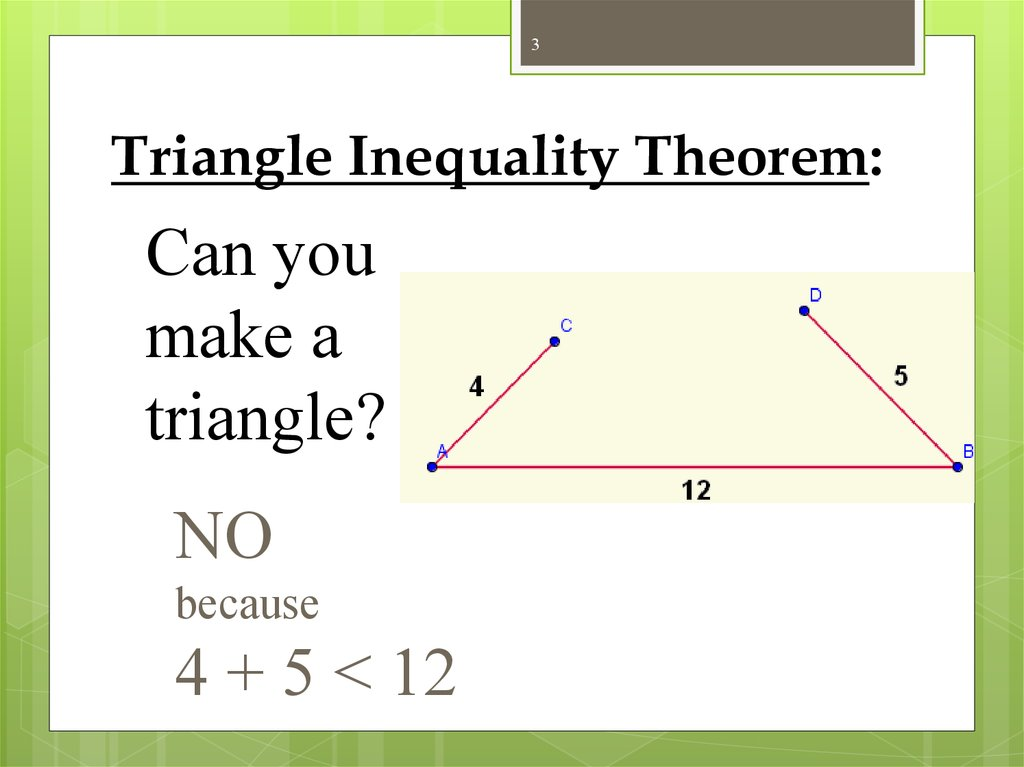# Triangle. Inequalities

## 1. G.5

Triangle
Inequalities
Modified by Lisa Palen
1

## 2. Triangle Inequality Theorem:

2
Triangle Inequality Theorem:
Can you
make a
triangle?
Yes!

## 3. Triangle Inequality Theorem:

3
Triangle Inequality Theorem:
Can you
make a
triangle?
NO
because
4 + 5 < 12

## 4. Triangle Inequality Theorem:

4
Triangle Inequality Theorem:
The sum of the lengths of any two
sides of a triangle is greater than
the length of the third side.
A
b
C
a+b>c
a+c>b
b+c>a
c
a
B

## 5. Finding the range of the third side:

5
Finding the range of the third side:
Example Given a triangle with sides of length 3 and 7, find
the range of possible values for the third side.
Solution Let x be the length of the third side of the triangle.
The maximum value:
x < 3 + 7 = 10
The minimum value:
x>7–3=4
So 4
x < 10
x>4
< x < 10 (x is between 4 and 10.)

## 6. Finding the range of the third side:

6
Finding the range of the third side:
Given The lengths of two sides of a triangle
Since the third side cannot be larger than the
other two added together, we find the
maximum value by adding the two sides.
Since the third side and the smallest side
given cannot be larger than the other side, we
find the minimum value by subtracting the
two sides.
Difference < Third Side < Sum

## 7. Finding the range of the third side:

7
Finding the range of the third side:
Example Given a triangle with sides of length a and b, find
the range of possible values for the third side.
Solution Let x be the length of the third side of the triangle.
The maximum value:
x<a+b
The minimum value:
x > |a – b|
x<a+b
x > |a – b|
|a – b|< x < a + b
(x is between |a – b| and a + b.)
So

## 8.

8
In a Triangle:
The smallest angle is opposite the smallest side.
The largest angle is opposite the largest side.
The smallest side is opposite the smallest angle.
The largest side is opposite the largest angle.
m CBA = 80 °
B
m BC = 4.1 cm
m AB = 6.3 cm
C m BCA = 64 °
m CA = 6.9 cm
A
m BAC = 36 °

## 9.

9
Theorem
•If one angle of a triangle is larger than a
second angle, then the side opposite the first
angle is larger than the side opposite the
second angle.
larger
angle B
shorter side
C
longer side
smaller angleA

## 10.

10
Theorem
•If one side of a triangle is larger than a
second side, then the angle opposite the first
side is larger than the angle opposite the
second side.

## 11. Corollary #1:

11
Corollary #1:
The perpendicular segment from a
point to a line is the shortest
segment from the point to the line.
This side is longer
because it is
opposite the
largest angle!
This is the
shortest segment!

## 12. Corollary #2:

12
Corollary #2:
The perpendicular segment from a
point to a plane is the shortest
segment from the point to the plane.
This side is longer
because it is
opposite the
largest angle!
This is the
shortest segment!